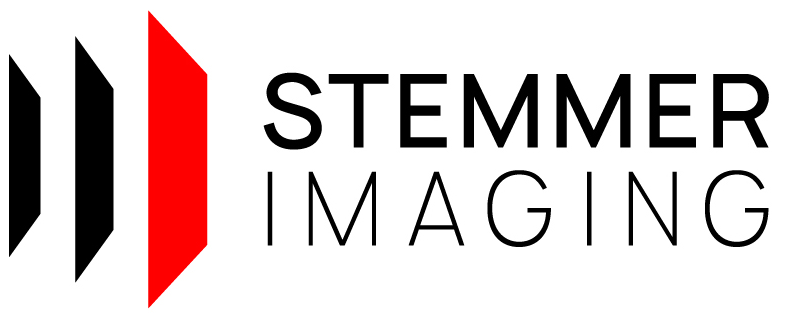CVB++ 14.0
Cvb::Foundation::Regression Namespace Reference

Various regression functions. More...

## Functions

template<class RANGE >
TypedRange< Circle, Point2D< double >, RANGE >::type CircleRegression (const RANGE &points)
Create a circle, that fits best into a collection of points. More...

template<class RANGE >
TypedRange< Ellipse, Point2D< double >, RANGE >::type EllipseRegression (const RANGE &points)
Create an ellipse, that fits best into a collection of points. More...

template<class RANGE >
TypedRange< Line2D, Point2D< double >, RANGE >::type LineRegression (const RANGE &points)
Create a line, that fits best into a collection of points. More...

## Detailed Description

Various regression functions.

Remarks
CMake users: Link to imported target CVB::CvbFoundationRegression

## ◆ CircleRegression()

 TypedRange< Circle, Point2D< double >, RANGE >::type CircleRegression ( const RANGE & points )
inline

Create a circle, that fits best into a collection of points.

Parameters
 [in] points Points to fit circle in.
Returns
Circle object.
Exceptions
 Any exception derived from std::exception including CvbException.

## ◆ EllipseRegression()

 TypedRange< Ellipse, Point2D< double >, RANGE >::type EllipseRegression ( const RANGE & points )
inline

Create an ellipse, that fits best into a collection of points.

Parameters
 [in] points Points to fit the ellipse in.
Returns
Ellipse object.
Exceptions
 Any exception derived from std::exception including CvbException.

## ◆ LineRegression()

 TypedRange< Line2D, Point2D< double >, RANGE >::type LineRegression ( const RANGE & points )
inline

Create a line, that fits best into a collection of points.

Parameters
 [in] points Points to fit line in.
Returns
Line2D object.
Exceptions
 Any exception derived from std::exception including CvbException.